# 按名字调用方法高级解决方案

### 时间：2011/9/3 15:37:21 点击：核心提示：名字调用方法似乎一直以来都是大家比较关注的技术，在论坛上有一个经典的答复：typeTProcedure = procedure(Test: string) of object;procedure Ex...

type
TProcedure = procedure(Test: string) of object;

procedure ExecuteRoutine(Obj: TObject; Name, Param: string);
var
PMethod: TMethod;
AProcedure: TProcedure;
begin
PMethod.Data := Pointer(Obj);
if Assigned(PMethod.Code) then
begin
AProcedure := TProcedure(PMethod);
AProcedure(Param);
end;
end;

使用：待调用方法声明为某个类的 published 方法，Obj 为拥有待调用方法的类的

procedure AProc(Param: array of const);

AProc([123, 'X', True, 'hello'...]);

type
TProcedure = procedure(Params: array of const) of object;

procedure ExecuteRoutine(Obj: TObject; Name: string; Params: array of const);
var
...
begin
...
AProcedure(Params);
...
end;

ExecuteRoutine 函数，为控制函数；待调用方法简称为待调方法）这个形参表的声明

TVarRec 的数组中），如何在调用它的时候将参数传进去。这需要几点预备知识：

1. 首先我们来看看传进来的这个参数表：Params。它的类型被 Delphi 称作可变开

Delphi 自动转化为了 TVarRec（参见帮助中的 Variant open array parameters 一

2. 调用函数时的参数传递的一般情况（未使用 stdcall 的情况）。对于一般的函数

3. 对于调用类的方法，其实有一个默认的隐藏参数 Self 作为第一个参数传入，放

4. 用 ObjectPascal 语法调用方法，Delphi 会自动帮我们处理参数的传递问题，而

function ExecuteRoutine(AObj: TObject; AName: string;
Params: array of const): DWord;
const
RecSize = SizeOf(TVarRec); // 循环处理参数列表时递增的字节数
var
PFunc: Pointer;
ParCount: DWord;
begin
if not Assigned(AObj) then
raise Exception.Create ('你确定传进来的是一个对象？');
if not Assigned(PFunc) then
raise Exception.CreateFmt('找不到 %s 的 Method: %s', [AObj.ClassName,
AName]);

ParCount := High(Params) + 1; // 获取参数个数

asm
PUSH        ESI                 // 保存 ESI，我们待会儿要用到它

MOV         ESI, Params         // ESI 指向参数表首址
CMP         ParCount, 1         // 判断参数个数
JB          @NoParam
JE          @OneParam
CMP         ParCount, 2
JE          @TwoParams

@ManyParams: // 超过两个参数
CLD                             // 清空方向标志
MOV         ECX, ParCount
SUB         ECX, 2              // 循环 ParCount - 2 次
MOV         EDX, RecSize        // EDX 依次指向每个参数的首址，每次递增 8 Bytes
@ParamLoop:
MOV         EAX, [ESI][EDX]     // 用基址变址寻址方式取得一个参数
PUSH        EAX                 // 参数进栈
ADD         EDX, RecSize        // EDX 指向下一个参数首址
LOOP        @ParamLoop

@TwoParams: // 两个参数
MOV         ECX, [ESI] + RecSize

@OneParam: // 一个参数
MOV         EDX, [ESI]

@NoParam:
MOV         EAX, AObj           // 传入实例地址（即，隐藏参数 Self）
CALL        PFunc               // 调用方法
MOV         Result, EAX         // 返回值放入 Result

POP         ESI                 // 记得还原
end;
end;

ShareMem，那不属于本文讨论的范畴），如果要返回一个长 string，请改为 PChar，

TForm1 = class(TForm)
Button1: TButton;
procedure Button1Click(Sender: TObject);
private
{ Private declarations }
public
{ Public declarations }
published // 几个待调方法
function TowInt(I, J: Integer): Integer;
function ThreeInt(I, J, K: Integer): Integer;
function FiveInt(X1, X2, X3, X4, X5: Integer): Integer;
function ThreeChar(I, J, K: Char): PChar;
function TwoStr(X, Y: string): PChar;
function IntNBool(I: Integer; B: Boolean): Boolean;
end;

...

function ExecuteRoutine(AObj: TObject; AName: string;
Params: array of const): DWord;
...

function TForm1.TowInt(I, J: Integer): Integer;
begin
ShowMessage(Format('%d + %d', [I, J]));
Result := I + J;
end;

function TForm1.ThreeInt(I, J, K: Integer): Integer;
begin
ShowMessage(Format('%d + %d + %d', [I, J, K]));
Result := I + J + K;
end;

function TForm1.FiveInt(X1, X2, X3, X4, X5: Integer): Integer;
begin
ShowMessage(Format('%d + %d + %d + %d + %d', [X1, X2, X3, X4, X5]));
Result := X1 + X2 + X3 + X4 + X5;
end;

function TForm1.ThreeChar(I, J, K: Char): PChar;
var
Res: string;
begin
ShowMessage(Format('%s + %s + %s', [I, J, K]));
Res := I + J + K;
Result := AllocMem(Length(Res) + 1);
StrPCopy(Result, Res);
end;

function TForm1.TwoStr(X, Y: string): PChar;
var
Res: string;
begin
ShowMessage(Format('%s + %s', [X, Y]));
Res := X + Y;
Result := AllocMem(Length(Res) + 1);
StrPCopy(Result, Res);
end;

function TForm1.IntNBool(I: Integer; B: Boolean): Boolean;
begin
if B then
ShowMessage(IntToStr(I) + ' and True')
else
ShowMessage(IntToStr(I) + ' and False');

Result := B;
end;

procedure TForm1.Button1Click(Sender: TObject);
var
i: Integer;
b: Boolean;
s: string;
begin
i := ExecuteRoutine(Self, 'ThreeInt', [10, 23, 17]);
ShowMessage('Result: ' + IntToStr(i));

i := ExecuteRoutine(Self, 'FiveInt', [1, 2, 3, 4, 5]);
ShowMessage('Result: ' + IntToStr(i));

b := Boolean(ExecuteRoutine(Self, 'IntNBool', [10, False]));
if b then
ShowMessage('Result: True')
else
ShowMessage('Result: False');

s := PChar(ExecuteRoutine(Self, 'ThreeChar', ['a', 'b', 'c']));
ShowMessage('Result: ' + s);

s := PChar(ExecuteRoutine(Self, 'TwoStr', ['hello', ' world']));
ShowMessage('Result: ' + s);
end;

...

###### 共有评论 0 条相关评论###### 发表我的评论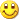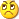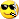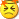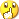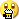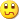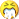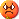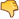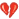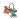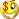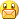• 大名：
• 内容：